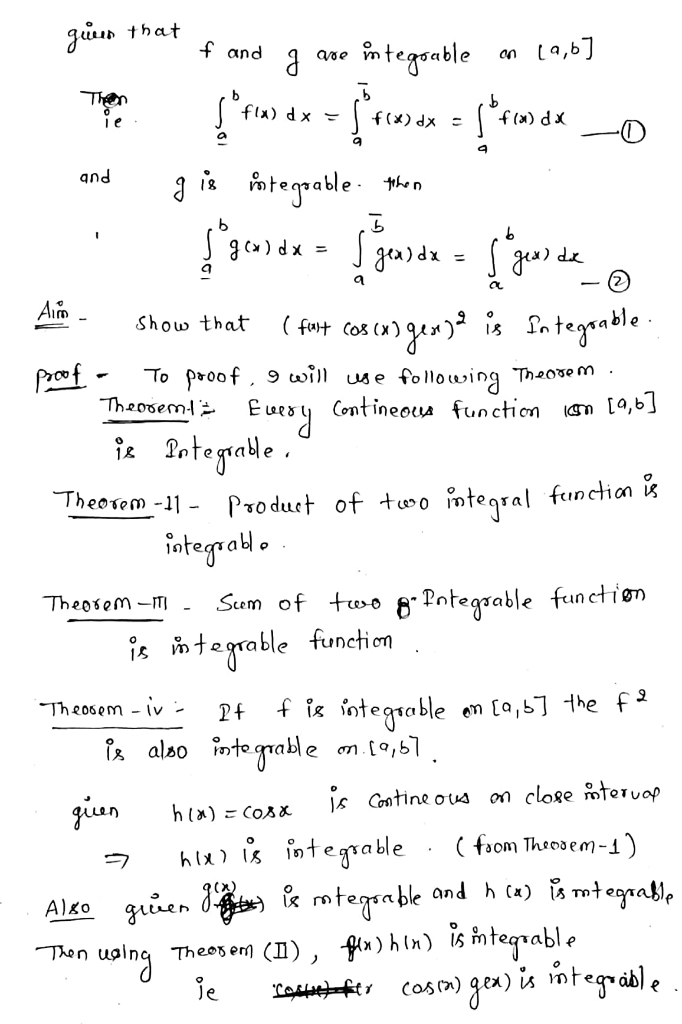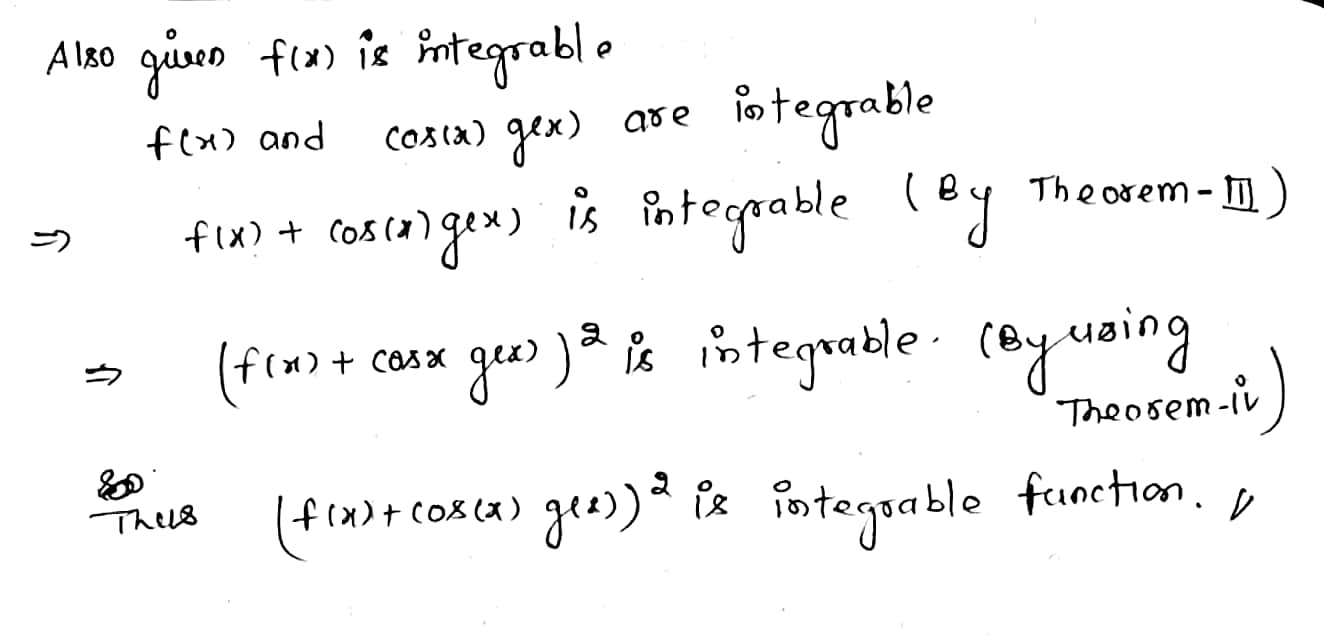# Prove that if f, g are integrable, then the function (f(x) + cos(x)g(x))2is integrable.

Prove that if f, g are integrable, then the function (f(x) + cos(x)g(x))2is integrable.

## Solutions

##### Expert Solution## Related Solutions

##### Let f: X→Y and g: Y→Z be both onto. Prove that g◦f is an onto function...
Let f: X→Y and g: Y→Z be both onto. Prove that g◦f is an onto function Let f: X→Y and g: Y→Z be both onto. Prove that f◦g is an onto function Let f: X→Y and g: Y→Z be both one to one. Prove that g◦f is an one to one function Let f: X→Y and g: Y→Z be both one to one. Prove that f◦g is an one to one function
##### prove using the definition of derivative that if f(x) and g(x) is differentiable than (f'(x)g(x) -...
prove using the definition of derivative that if f(x) and g(x) is differentiable than (f'(x)g(x) - f(x)g'(x))/g^2(x)
##### Prove that a continuous function for sections(subrectangles) is integrable in R^N
Prove that a continuous function for sections(subrectangles) is integrable in R^N
##### Consider a continuous, integrable, twice-differentiable function f with input variable x. In terms of the units...
Consider a continuous, integrable, twice-differentiable function f with input variable x. In terms of the units of f and the units of x, choose the units of each function or expression below: (a) The units of f ' are the units of f the units of x      (the units of f)(the units of x ) the units of f the units of x    the units of f (the units of x)2    the units of f (the units...
##### True and False (No need to solve). 1. Every bounded continuous function is integrable. 2. f(x)=|x|...
True and False (No need to solve). 1. Every bounded continuous function is integrable. 2. f(x)=|x| is not integrable in [-1, 1] because the function f is not differentiable at x=0. 3. You can always use a bisection algorithm to find a root of a continuous function. 4. Bisection algorithm is based on the fact that If f is a continuous function and f(x1) and f(x2) have opposite signs, then the function f has a root in the interval (x1,...
##### For the given function determine the following: f (x) = (sin x + cos x) 2...
For the given function determine the following: f (x) = (sin x + cos x) 2 ; [−π,π] a) Find the intervals where f(x) is increasing, and decreasing b) Find the intervals where f(x) is concave up, and concave down c) Find the x-coordinate of all inflection points
##### The function F(x) = x2 - cos(π x) is defined on the interval 0 ≤ x...
The function F(x) = x2 - cos(π x) is defined on the interval 0 ≤ x ≤ 1 radians. Explain how the Intermediate Value Theorem shows that F(x) = 0 has a solution on the interval 0 < x < .
##### 2. Let f(x) ≥ 0 on [1, 2] and suppose that f is integrable on [1,...
2. Let f(x) ≥ 0 on [1, 2] and suppose that f is integrable on [1, 2] with R 2 1 f(x)dx = 2 3 . Prove that f(x 2 ) is integrable on [1, √ 2] and √ 2 6 ≤ Z √ 2 1 f(x 2 )dx ≤ 1 3 .
##### (a) State the definition of the derivative of f. (b) Using (a), prove the following:d/dx(f(x) +g(x))...
(a) State the definition of the derivative of f. (b) Using (a), prove the following:d/dx(f(x) +g(x)) =d/dx(f(x)) +d/dx(g(x))
##### Consider the function on the interval (0, 2π). f(x) = sin(x) cos(x) + 2 (a) Find...
Consider the function on the interval (0, 2π). f(x) = sin(x) cos(x) + 2 (a) Find the open interval(s) on which the function is increasing or decreasing. (Enter your answers using interval notation.) increasing     ( )    decreasing     ( )   (b) Apply the First Derivative Test to identify all relative extrema. relative maxima     (x, y) =    (smaller x-value) (x, y) = ( )    (larger x-value) relative minima (x, y) =    (smaller x-value) (x, y) = ​   ...